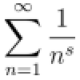Ngô Quốc Anh

September 1, 2010

The inverse of the Laplace transform by contour integration

Filed under: Giải tích 7 (MA4247) — Ngô Quốc Anh @ 17:23

Usually, we can find the inverse of the Laplace transform$\mathcal L[\cdot](s)$ by looking it up in a table. In this entry, we show an alternative method that inverts Laplace transforms through the powerful method of contour integration.

Consider the piece-wise differentiable function$f(x)$ that vanishes for$x < 0$. We can express the function$e^{-cx}f(x)$ by the complex Fourier representation of$\displaystyle f(x){e^{ - cx}} = \frac{1}{{2\pi }}\int_{ - \infty }^\infty {{e^{i\omega x}}\left[ {\int_0^\infty {{e^{ - ct}}f(t){e^{ - i\omega t}}dt} } \right]d\omega }$

for any value of the real constant$c$, where the integral$\displaystyle I = \int_0^\infty {{e^{ - ct}}|f(t)|dt}$

exists. By multiplying both sides of first equation by$e^{cx}$ and bringing it inside the first integral$\displaystyle f(x) = \frac{1}{{2\pi }}\int_{ - \infty }^\infty {{e^{(c + i\omega )x}}\left[ {\int_0^\infty {f(t){e^{ - (c + i\omega )t}}dt} } \right]d\omega }$.

With the substitution$z = c+\omega i$, where$z$ is a new, complex variable of integration,$\displaystyle f(x) = \frac{1}{{2\pi }}\int_{c - \infty i}^{c + \infty i} {{e^{zx}}\left[ {\int_0^\infty {f(t){e^{ - zt}}dt} } \right]d\omega }$.

The quantity inside the square brackets is the Laplace transform$\mathcal L[f](z)$. Therefore, we can express$f(t)$ in terms of its transform by the complex contour integral

Theorem (Bromwich’s integral). The following line integral runs along the line$x = c$ parallel to the imaginary axis and$c$ units to the right of it, the so-called Bromwich contour$\displaystyle f(t) = \frac{1}{{2\pi i}}\int_{c - \infty i}^{c + \infty i} {\mathcal L[f](z){e^{tz}}dz}$.

Example. Let us invert$\displaystyle L(s) = \frac{{{e^{ - 3s}}}}{{{s^2}(s - 1)}}$.

Solution. From Bromwich’s integral,$\displaystyle\begin{gathered} f(t) = \frac{1}{{2\pi i}}\int_{c - \infty i}^{c + \infty i} {\frac{{{e^{(t - 3)s}}}}{{{z^2}(z - 1)}}dz} \hfill \\ \qquad= \frac{1}{{2\pi i}}\oint_C {\frac{{{e^{(t - 3)s}}}}{{{z^2}(z - 1)}}dz} - \frac{1}{{2\pi i}}\int_{{C_R}} {\frac{{{e^{(t - 3)s}}}}{{{z^2}(z - 1)}}dz} \hfill \\ \end{gathered}$

where$C_R$ is a semicircle of infinite radius in either the right or left half of the$z$-plane and$C$ is the closed contour that includes$C_R$ and Bromwich’s contour.

Our first task is to choose an appropriate contour so that the integral along$C_R$ vanishes. By Jordan’s lemma, this requires a semicircle in the right half-plane if$t-3 < 0$ and a semicircle in the left half-plane if$t- 3>0$. Consequently, by considering these two separate cases, we force the second integral to zero and the inversion simply equals the closed contour.

Consider the case$t < 3$ first. Because Bromwich’s contour lies to the right of any singularities, there are no singularities within the closed contour and$f(t) = 0$.

Consider now the case$t > 3$. Within the closed contour in the left half-plane, there is a second-order pole at$z = 0$ and a simple pole at$z = 1$. Therefore,$\displaystyle f(t) = \mathop {\rm Res}\limits_{z = 0} \left[ {\frac{{{e^{(t - 3)s}}}}{{{z^2}(z - 1)}}} \right] + \mathop {\rm Res}\limits_{z = 1} \left[ {\frac{{{e^{(t - 3)s}}}}{{{z^2}(z - 1)}}} \right]$

where$\displaystyle\mathop {\rm Res}\limits_{z = 0} \left[ {\frac{{{e^{(t - 3)s}}}}{{{z^2}(z - 1)}}} \right] = \mathop {\lim }\limits_{z \to 0} \frac{d}{{dz}}\left[ {{z^2}\frac{{{e^{(t - 3)s}}}}{{{z^2}(z - 1)}}} \right] = 2 - t$

and$\displaystyle\mathop {\rm Res}\limits_{z = 1} \left[ {\frac{{{e^{(t - 3)s}}}}{{{z^2}(z - 1)}}} \right] = \mathop {\lim }\limits_{z \to 1} \frac{d}{{dz}}\left[ {(z - 1)\frac{{{e^{(t - 3)s}}}}{{{z^2}(z - 1)}}} \right] = {e^{t - 3}}$.

Taking our earlier results into account, the inverse equals$\displaystyle f(t) = \left[ {{e^{t - 3}} - (t - 3) - 1} \right]H(t - 3)$.

Source: Green’s Functions with Applications by Dean G. Duffy

1.Excuse me, I have a question.
the first equality on the solution of the example, I think z have to be put instead of s.
like this$\displaystyle f(t) = \frac{1}{2\pi i}\int_{c-\infty i}^{c+\infty i}\frac{e^{(t-3)z}}{z^2 (z-1)}dz$
Am I right?

Comment by zariski — September 10, 2010 @ 19:41

•Hi zariski.

You are right, it also appears that$s$ in the second line need to be changed, i.e., the whole stuff reads as follows$\displaystyle\begin{gathered} f(t) = \frac{1}{{2\pi i}}\int_{c - \infty i}^{c + \infty i} {\frac{{{e^{(t - 3)z}}}}{{{z^2}(z - 1)}}dz} \hfill \\ \qquad= \frac{1}{{2\pi i}}\oint_C {\frac{{{e^{(t - 3)z}}}}{{{z^2}(z - 1)}}dz} - \frac{1}{{2\pi i}}\int_{{C_R}} {\frac{{{e^{(t - 3)z}}}}{{{z^2}(z - 1)}}dz}. \hfill \\ \end{gathered}$

Thanks for visiting my blog and also pointing out the misprint.

Comment by Ngô Quốc Anh — September 10, 2010 @ 21:27

2.Thanks for this post. It was a useful overview.

Comment by Sunil — August 12, 2012 @ 9:10

This site uses Akismet to reduce spam. Learn how your comment data is processed.